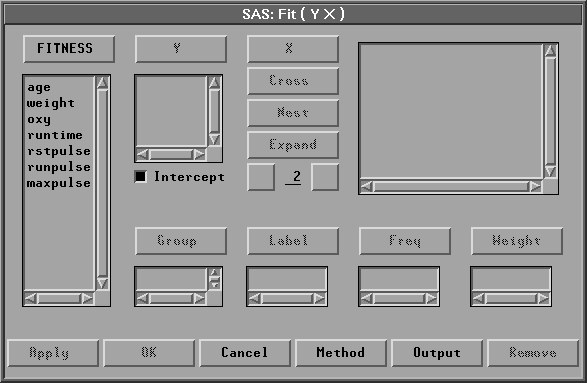Fit Analyses

# Variables

To create a fit analysis, choose Analyze:Fit ( Y X ). If you have already selected one or more variables, the first variable selected is the response or dependent variable, and it is assigned the Y variable role. The remaining variables are explanatory or independent variables, and they are assigned the X variable role. If you do not select any X effects, a model with only an intercept term (mean) is fit.

If you have not selected any variables, a variables dialog appears.Figure 39.2: Fit Variables Dialog

In the dialog, select one Y variable for each fit analysis. Create X effects in the model by using the X, Cross, Nest, and Expand buttons. An effect is a variable or combination of variables that constitutes a term in the model. There are four ways to specify effects in SAS/INSIGHT software. In the following discussion, assume that X1 and X2 are interval variables and A and B are nominal variables.

You can use the X button to create regressor effects of the interval variables and main effects of the nominal variables. Select any variable, then click the X button. For example, selecting A and then clicking the X button adds A to the effects list.

You can use the Cross button to create crossed effects. These include polynomial effects of the interval variables, interactions of the nominal variables, and interaction effects of interval and nominal variables. Select two or more variables, then click the Cross button. For example, selecting X1 and X2 and then clicking the Cross button generates the crossed effect X1*X2.

You can use the Nest button to create nested effects. In a nested effect, a variable or crossed effect is nested within the effects of one or more nominal variables. Select a variable or crossed effect and one or more nominal variables, then click the Nest button. For example, selecting X1*X2, A, and B and then clicking the Nest button generates the nested effect X1*X2(A B).

You can use the Expand button and the associated entry field to create expanded effects. These include response surface effects for interval variables and factorial effects for nominal variables. The Expand button expands all possible effects to the degree of expansion specified in the entry field below the Expand button. The value 2 is the default degree of expansion. You can click the right button of the entry field to increase the expansion degree by 1 or the left button to decrease it by 1.

Choose the degree of expansion, then select variables or effects and click the Expand button. For example, with degree of expansion 2 and variables A and B selected, clicking the Expand button generates three effects

A       B       A*B

With degree of expansion 2 and variables X1 and X2 selected, clicking the Expand button generates five effects

X1       X2      X1*X1       X1*X2       X2*X2

Intercept is checked by default to include the intercept term in the model. As a general rule, no-intercept models should be fit only when theoretical justification exists.

You can select one or more Group variables if you have grouped data. This creates a fit analysis for each group.

You can select a Label variable to label observations in the plots.

You can select a Freq variable. If you select a Freq variable, each observation is assumed to represent n observations, where n is the value of the Freq variable.

You can select a Weight variable to assign relative weights for each observation in the analysis. The details of weighted analyses are explained in the "Weighted Analyses" section at the end of this chapter.

The fit variables dialog provides an Apply button. The Apply button displays the fit window without closing the fit variables dialog. This makes it easy to modify the model by adding or removing variables. Each time you modify the model using the Apply button, a new fit window is displayed so you can easily compare models. The OK button also displays a new fit window but closes the dialog.

Copyright © 2007 by SAS Institute Inc., Cary, NC, USA. All rights reserved.